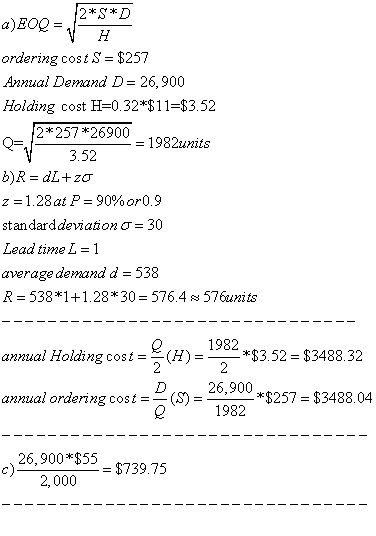# Answered! Given the following information, formulate an inventory management system. The item is demanded 50 weeks a year….

 Given the following information, formulate an inventory management system. The item is demanded 50 weeks a year.
 Item cost \$ 11.00 Standard deviation of weekly demand 30 per week Order cost \$ 257.00 Lead time 1 week Annual holding cost (%) 32 % of item cost Service probability 90 % Annual demand 26,900 Average demand 538 per week
 a. Determine the order quantity and reorder point. (Use Excel’s NORMSINV( ) function to find your z-value and then round that z-value to 2 decimal places. Do not round any other intermediate calculations. Round your final answers to the nearest whole number.)
 Optimal order quantity units Reorder point units
 b. Determine the annual holding and order costs. (Do not round any intermediate calculations. Round your final answers to 2 decimal places.)
 Holding cost \$ Ordering cost \$
 c. Assume a price break of \$55 per order was offered for purchase quantities of 2,000 units per order. If you took advantage of this price break, how much would you save annually? (Do not round any intermediate calculations (including number of setups per year). Round your final answer to 2 decimal places.)
 Annual savings \$